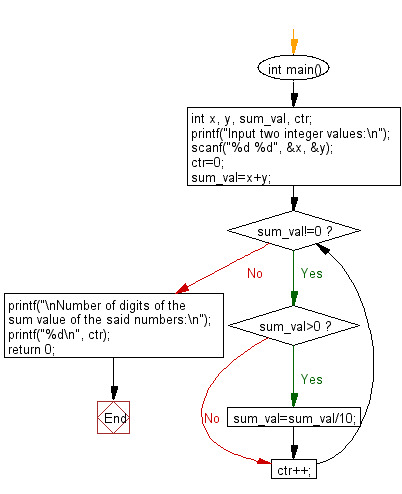﻿ C : Number of digits of the sum value of two integer

# C Exercises: Count the number of digits of the sum value of two integer values

## C Basic Declarations and Expressions: Exercise-133 with Solution

Write a C program to calculate the sum of two given integers and count the number of digits in the sum value.

Sample Solution:

C Code:

``````#include <stdio.h>
int main()
{
int x, y, sum_val, ctr;
printf("Input two integer values:\n");
scanf("%d %d", &x, &y);

ctr = 0; // Initialize a counter to keep track of digits
sum_val = x + y; // Calculate the sum of x and y

// Loop to count the number of digits in the sum value
while (sum_val != 0)
{
if (sum_val > 0)
{
sum_val = sum_val / 10; // Divide by 10 to remove the rightmost digit
}
ctr++; // Increment the counter
}

// Print the number of digits in the sum value
printf("\nNumber of digits of the sum value of the said numbers:\n");
printf("%d\n", ctr);

return 0; // End of program
}
``````

Sample Output:

```Input two integer values:
68
75

Number of digits of the sum value of the said numbers:
3
```

Flowchart:C programming Code Editor:

What is the difficulty level of this exercise?

Test your Programming skills with w3resource's quiz.

﻿